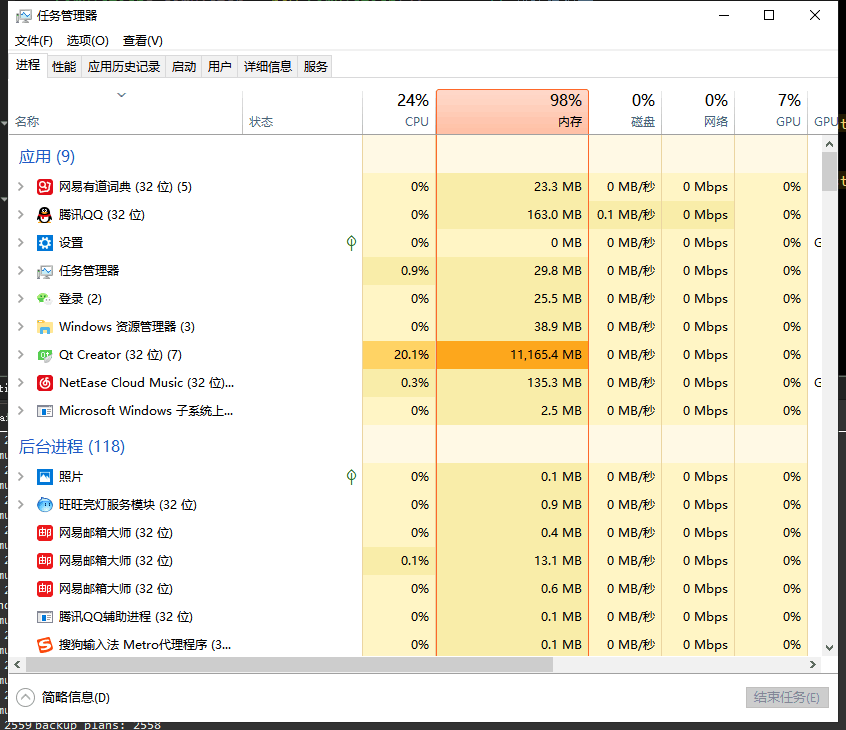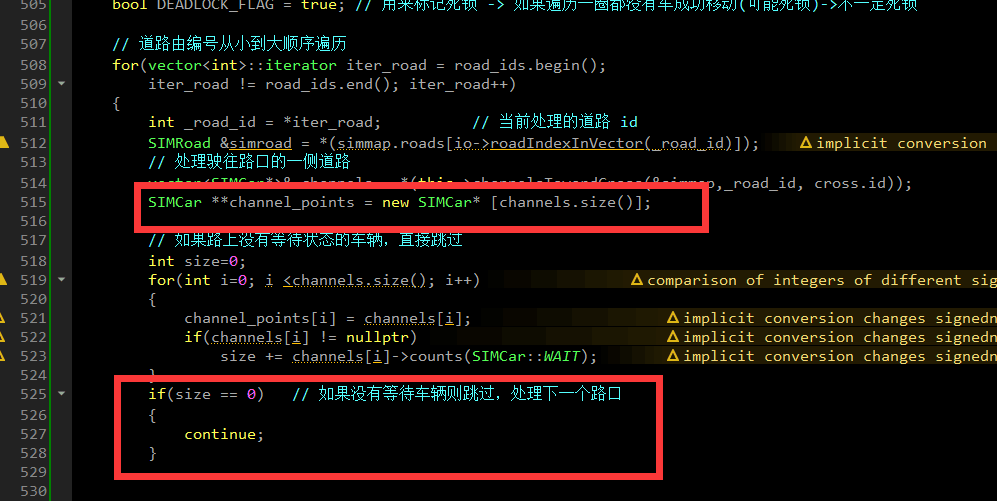# C++ 智能指针--内存泄露的惨痛经历

layout: post
title: “C++ 指针”
subtitle: “避免 C++ 内存泄露的惨痛经历”
date: 2019-04-1
author: Mcoder
header-img: img/JCQ_0383-Pano.jpg
catalog: true
tags:

• C++
• memory
• Smart Pointer

# 引言# 查询内存泄露方法

## 啥是内存泄露

• 程序运行后，随着时间占用了更多的内存，最后无内存可用而崩溃；
• 程序消耗了大量的内存，导致其他程序无法正常使用；
• 程序消耗了大量内存，导致消费者选用了别人的程序而不是你的；
• 经常做出内存泄露bug的程序猿被公司开出而贫困潦倒。

## 如何定位到泄露点呢？

1. 根据原理，我们可以先review自己的代码，利用"查找"功能，查询newdelete，看看内存的申请与释放是不是成对释放的，这使你迅速发现一些逻辑较为简单的内存泄露情况。

2. 如果依旧发生内存泄露，可以通过记录申请与释放的对象数目是否一致来判断。在类中追加一个静态变量 static int count;在构造函数中执行count++;在析构函数中执行count--;，通过在程序结束前将所有类析构，之后输出静态变量，看count的值是否为0，如果为0,则问题并非出现在该处，如果不为0，则是该类型对象没有完全释放。

3. 检查类中申请的空间是否完全释放，尤其是存在继承父类的情况，看看子类中是否调用了父类的析构函数，有可能会因为子类析构时没有是否父类中申请的内存空间。

4. 对于函数中申请的临时空间，认真检查，是否存在提前跳出函数的地方没有释放内存。

# STL 的智能指针

unique_ptr C++ 11 拥有独有对象所有权语义的智能指针
shared_ptr C++ 11 拥有共享对象所有权语义的智能指针
weak_ptr C++ 11 到 std::shared_ptr 所管理对象的弱引用
auto_ptr C++ 17中移除 拥有严格对象所有权语义的智能指针

std::unique_ptr 是通过指针占有并管理另一对象，并在 unique_ptr 离开作用域时释放该对象的智能指针。在下列两者之一发生时用关联的删除器释放对象：

• 销毁了管理的 unique_ptr 对象
• 通过 operator= 或 reset() 赋值另一指针给管理的 unique_ptr 对象。

std::shared_ptr 是通过指针保持对象共享所有权的智能指针。多个 shared_ptr 对象可占有同一对象。下列情况之一出现时销毁对象并解分配其内存：

• 最后剩下的占有对象的 shared_ptr 被销毁；
• 最后剩下的占有对象的 shared_ptr 被通过 operator= 或 reset() 赋值为另一指针。

## unique_ptr

### 创建

unique_ptr<int> uptr( new int );
unique_ptr<int[ ]> uptr( new int );

//声明,可以用一个指针显示的初始化,或者声明成一个空指针,可以指向一个类型为T的对象
shared_ptr<T> sp;
unique_ptr<T> up;
//赋值,返回相对应类型的智能指针,指向一个动态分配的T类型对象,并且用args来初始化这个对象
make_shared<T>(args);
make_unique<T>(args);     //注意make_unique是C++14之后才有的
//用来做条件判断,如果其指向一个对象,则返回true否则返回false
p;
//解引用
*p;
//获得其保存的指针,一般不要用
p.get();
//交换指针
swap(p,q);
p.swap(q);

//release()用法
//release()返回原来智能指针指向的指针,只负责转移控制权，不负责释放内存，常见的用法
unique_ptr<int> q(p.release()) // 此时p失去了原来的的控制权交由q,同时p指向nullptr
//所以如果单独用:
p.release()
//则会导致p丢了控制权的同时,原来的内存得不到释放
//则会导致//reset()用法
p.reset()     // 释放p原来的对象,并将其置为nullptr，
p = nullptr   // 等同于上面一步
p.reset(q)    // 注意此处q为一个内置指针,令p释放原来的内存，p新指向这个对象



### Demo

#include <iostream>
#include <vector>
#include <memory>
#include <cstdio>
#include <fstream>
#include <cassert>
#include <functional>

struct B {
virtual void bar() { std::cout << "B::bar\n"; }
virtual ~B() = default;
};
struct D : B
{
D() { std::cout << "D::D\n";  }
~D() { std::cout << "D::~D\n";  }
void bar() override { std::cout << "D::bar\n";  }
};

// 消费 unique_ptr 的函数能以值或以右值引用接收它
std::unique_ptr<D> pass_through(std::unique_ptr<D> p)
{
p->bar();
return p;
}

void close_file(std::FILE* fp) { std::fclose(fp); }

int main()
{
std::cout << "unique ownership semantics demo\n";
{
auto p = std::make_unique<D>(); // p 是占有 D 的 unique_ptr
auto q = pass_through(std::move(p));
assert(!p); // 现在 p 不占有任何内容并保有空指针
q->bar();   // 而 q 占有 D 对象
} // ~D 调用于此

std::cout << "Runtime polymorphism demo\n";
{
std::unique_ptr<B> p = std::make_unique<D>(); // p 是占有 D 的 unique_ptr
// 作为指向基类的指针
p->bar(); // 虚派发

std::vector<std::unique_ptr<B>> v;  // unique_ptr 能存储于容器
v.push_back(std::make_unique<D>());
v.push_back(std::move(p));
v.emplace_back(new D);
for(auto& p: v) p->bar(); // 虚派发
} // ~D called 3 times

std::cout << "Custom deleter demo\n";
std::ofstream("demo.txt") << 'x'; // 准备要读的文件
{
std::unique_ptr<std::FILE, void (*)(std::FILE*) > fp(std::fopen("demo.txt", "r"),
close_file);
if(fp) // fopen 可以打开失败；该情况下 fp 保有空指针
std::cout << (char)std::fgetc(fp.get()) << '\n';
} // fclose() 调用于此，但仅若 FILE* 不是空指针
// （即 fopen 成功）

std::cout << "Custom lambda-expression deleter demo\n";
{
std::unique_ptr<D, std::function<void(D*)>> p(new D, [](D* ptr)
{
std::cout << "destroying from a custom deleter...\n";
delete ptr;
});  // p 占有 D
p->bar();
} // 调用上述 lambda 并销毁 D

std::cout << "Array form of unique_ptr demo\n";
{
std::unique_ptr<D[]> p{new D};
} // 调用 ~D 3 次
}


unique ownership semantics demo
D::D
D::bar
D::bar
D::~D
Runtime polymorphism demo
D::D
D::bar
D::D
D::D
D::bar
D::bar
D::bar
D::~D
D::~D
D::~D
Custom deleter demo
x
Custom lambda-expression deleter demo
D::D
D::bar
destroying from a custom deleter...
D::~D
Array form of unique_ptr demo
D::D
D::D
D::D
D::~D
D::~D
D::~D


## shared_ptr


void main( )
{
shared_ptr<int> sptr1( new int );
shared_ptr<int> sptr2 = make_shared<int>(100);
}


### 析构

shared_ptr默认调用delete释放关联的资源。如果用户采用一个不一样的析构策略时，他可以自由指定构造这个shared_ptr的策略。在此场景下，shared_ptr指向一组对象，但是当离开作用域时，默认的析构函数调用delete释放资源。实际上，我们应该调用delete[]来销毁这个数组。用户可以通过调用一个函数，例如一个lamda表达式，来指定一个通用的释放步骤。

void main( )
{
shared_ptr<Test> sptr1( new Test,
[ ](Test* p) { delete[ ] p; } );
}


void main( )
{
// 错误
int* p = new int;
shared_ptr<int> sptr1( p);   // count 1
shared_ptr<int> sptr2( p );  // count 1

// 正确
shared_ptr<int> sptr1( new int );  // count 1
shared_ptr<int> sptr2 = sptr1;     // count 2
shared_ptr<int> sptr3;
sptr3 =sptr1                       // count 3
}


### 循环引用

class B;
class A
{
public:
A(  ) : m_sptrB(nullptr) { };
~A( )
{
cout<<" A is destroyed"<<endl;
}
shared_ptr<B> m_sptrB;
};
class B
{
public:
B(  ) : m_sptrA(nullptr) { };
~B( )
{
cout<<" B is destroyed"<<endl;
}
shared_ptr<A> m_sptrA;
};
//***********************************************************
void main( )
{
shared_ptr<B> sptrB( new B );  // sptB count 1
shared_ptr<A> sptrA( new A );  // sptB count 1
sptrB->m_sptrA = sptrA;    // sptB count 2
sptrA->m_sptrB = sptrB;    // sptA count 2
}

// 超出定义域
// sptA count 1
// sptB count 2


### demo

#include <iostream>
#include <memory>
#include <thread>
#include <chrono>
#include <mutex>

struct Base
{
Base() { std::cout << "  Base::Base()\n"; }
// 注意：此处非虚析构函数 OK
~Base() { std::cout << "  Base::~Base()\n"; }
};

struct Derived: public Base
{
Derived() { std::cout << "  Derived::Derived()\n"; }
~Derived() { std::cout << "  Derived::~Derived()\n"; }
};

void thr(std::shared_ptr<Base> p)
{
std::this_thread::sleep_for(std::chrono::seconds(1));
std::shared_ptr<Base> lp = p; // 线程安全，虽然自增共享的 use_count
{
static std::mutex io_mutex;
std::lock_guard<std::mutex> lk(io_mutex);
std::cout << "local pointer in a thread:\n"
<< "  lp.get() = " << lp.get()
<< ", lp.use_count() = " << lp.use_count() << '\n';
}
}

int main()
{
std::shared_ptr<Base> p = std::make_shared<Derived>();

std::cout << "Created a shared Derived (as a pointer to Base)\n"
<< "  p.get() = " << p.get()
<< ", p.use_count() = " << p.use_count() << '\n';
std::thread t1(thr, p), t2(thr, p), t3(thr, p);
p.reset(); // 从 main 释放所有权
std::cout << "Shared ownership between 3 threads and released\n"
<< "ownership from main:\n"
<< "  p.get() = " << p.get()
<< ", p.use_count() = " << p.use_count() << '\n';
t1.join(); t2.join(); t3.join();
std::cout << "All threads completed, the last one deleted Derived\n";
}


Base::Base()
Derived::Derived()
Created a shared Derived (as a pointer to Base)
p.get() = 0xc99028, p.use_count() = 1
Shared ownership between 3 threads and released
ownership from main:
p.get() = (nil), p.use_count() = 0
local pointer in a thread:
lp.get() = 0xc99028, lp.use_count() = 3
local pointer in a thread:
lp.get() = 0xc99028, lp.use_count() = 4
local pointer in a thread:
lp.get() = 0xc99028, lp.use_count() = 2
Derived::~Derived()
Base::~Base()
All threads completed, the last one deleted Derived


## weak_ptr

std::weak_ptr 是一种智能指针，它对被 std::shared_ptr 管理的对象存在非拥有性（“弱”）引用。在访问所引用的对象前必须先转换为 std::shared_ptr。

std::weak_ptr 用来表达临时所有权的概念：当某个对象只有存在时才需要被访问，而且随时可能被他人删除时，可以使用 std::weak_ptr 来跟踪该对象。需要获得临时所有权时，则将其转换为 std::shared_ptr，此时如果原来的 std::shared_ptr 被销毁，则该对象的生命期将被延长至这个临时的 std::shared_ptr 同样被销毁为止。

std::weak_ptr 的另一用法是打断 std::shared_ptr 所管理的对象组成的环状引用。若这种环被孤立（例如无指向环中的外部共享指针），则 shared_ptr 引用计数无法抵达零，而内存被泄露。能令环中的指针之一为弱指针以避免此情况。

### 创建

void main( )
{
shared_ptr<Test> sptr( new Test );   // 强引用 1
weak_ptr<Test> wptr( sptr );         // 强引用 1 弱引用 1
weak_ptr<Test> wptr1 = wptr;         // 强引用 1 弱引用 2
}


• 调用use-count()去获取引用计数，该方法只返回强引用计数，并不返回弱引用计数。
• 调用expired()方法。比调用use_count()方法速度更快。

### 解决 shared_ptr 循环引用问题

class B;
class A
{
public:
A(  ) : m_a(5)  { };
~A( )
{
cout<<" A is destroyed"<<endl;
}
void PrintSpB( );
weak_ptr<B> m_sptrB;
int m_a;
};
class B
{
public:
B(  ) : m_b(10) { };
~B( )
{
cout<<" B is destroyed"<<endl;
}
weak_ptr<A> m_sptrA;
int m_b;
};

void A::PrintSpB( )
{
if( !m_sptrB.expired() )
{
cout<< m_sptrB.lock( )->m_b<<endl;
}
}

void main( )
{
shared_ptr<B> sptrB( new B );
shared_ptr<A> sptrA( new A );
sptrB->m_sptrA = sptrA;
sptrA->m_sptrB = sptrB;
sptrA->PrintSpB( );
}


# STL 智能指针的陷阱/不够智能的地方

1. 尽量用make_shared/make_unique，少用new

std::shared_ptr在实现的时候使用的refcount技术，因此内部会有一个计数器（控制块，用来管理数据）和一个指针，指向数据。因此在执行std::shared_ptr<A> p2(new A) 的时候，首先会申请数据的内存，然后申请内控制块，因此是两次内存申请，而std::make_shared<A>()则是只执行一次内存申请，将数据和控制块的申请放到一起。

1. 不要使用相同的内置指针来初始化(或者reset)多个智能指针

2. 不要delete get()返回的指针

3. 不要用get()初始化/reset另一个智能指针

4. 智能指针管理的资源它只会默认删除new分配的内存,如果不是new分配的则要传递给其一个删除器

5. 不要把this指针交给智能指针管理

以下代码发生了什么事情呢？还是同样的错误。把原生指针 this 同时交付给了 m_sp 和 p 管理，这样会导致 this 指针被 delete 两次。
这里值得注意的是：以上所说的交付给m_sp 和 p 管理不对，并不是指不能多个shared_ptr同时占有同一类资源。shared_ptr之间的资源共享是通过shared_ptr智能指针拷贝、赋值实现的，因为这样可以引起计数器的更新；而如果直接通过原生指针来初始化，就会导致m_sp和p都根本不知道对方的存在，然而却两者都管理同一块地方。相当于”一间庙里请了两尊神”。

class Test{
public:
void Do(){  m_sp =  shared_ptr<Test>(this);  }
private:
shared_ptr<Test> m_sp;
};
int main()
{
Test* t = new Test;
shared_ptr<Test> p(t);
p->Do();
return 0;
}

6. 不要把一个原生指针给多个shared_ptr或者unique_ptr管理

C++
int* ptr = new int;
shared_ptr<int> p1(ptr);
shared_ptr<int> p2(ptr);
//p1,p2析构的时候都会释放ptr，同一内存被释放多次！


1. 不是使用new出来的空间要自定义删除器

C++
int main()
{
int* pi = (int*)malloc(4 * sizeof(int));
shared_ptr<int> sp(pi);
return 0;
}


1. 尽量不要使用 get()

int main()
{
shared_ptr<int> sp(new int(4));
shared_ptr<int> pp(sp.get());
return 0;
}